Courses

# Trusses Civil Engineering Civil Engineering (CE) Notes | EduRev

## Topic wise GATE Past Year Papers for Civil Engineering

Created by: Gate Gurus

## Civil Engineering (CE) : Trusses Civil Engineering Civil Engineering (CE) Notes | EduRev

The document Trusses Civil Engineering Civil Engineering (CE) Notes | EduRev is a part of the Civil Engineering (CE) Course Topic wise GATE Past Year Papers for Civil Engineering.
All you need of Civil Engineering (CE) at this link: Civil Engineering (CE)

Q.1 Consider the pin-jointed plane truss shown in figure (not drawn to scale). Let RP, RQ, and RR denote the vertical reactions (upward positive) applied by the supports at P, Q and R, respectively, on the truss. The correct combination of (RP, RQ, RR) is represented by    [2019 : 1 Mark, Set-I]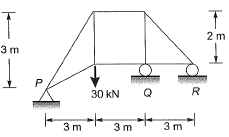(a) (10, 30,-10) kN
(b) (30,-30, 30) kN
(c) (20, 0,10) kN
(d) (0,60,-30) kN
Ans.
(B)
Solution: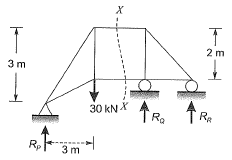Using,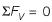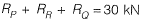...(i)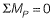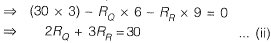Again considering the equilibrium of RHS of section X-X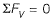∴ RR=-RQ ...(iii)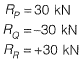Q.2 All the members of the planar truss (see figure), have the same properties in terms of area of cross-section (A) and modulus of elasticity (E),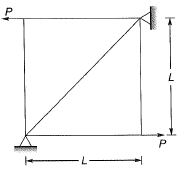For the loads shown on the truss, the statement that correctly represents the nature of forces in the members of the truss is:    [2018 : 1 Mark, Set-II]
(a) There are 3 members in tension, and 2 members in compression
(b) There are 2 members in tension, 2 members in compression, and 1 zero-force member
(c) There are 2 members in tension, 1 member in compression, and 2 zero-force members
(d) There are 2 members in tension, and 3 zero- force Members
Ans.
(D)
Solution: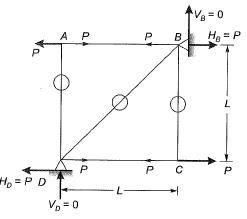Since member BD neither elongate nor contract.
Hence, FBD 0
So there are 2 tension members (AB and DC) and 3 zero force members (AD, BD, BC).

Q.3 Consider the deformable pin-jointed truss with loading, geometry and section properties as shown in figure.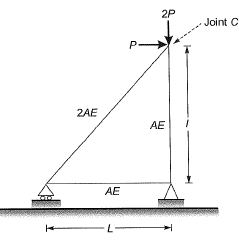Given that E = 2 x 1011 N/m2, A = 10 mm2, L = 1 m and P= 1 kN. the horizontal displacement of Joint C (in mm, up to one decimal place) is_____    [2018 : 2 Marks, Set-I]
Solution: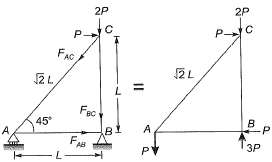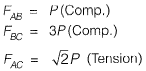Force in each member due to unit load.

Q.4 Consider the structural system shown in the figure under the action of weight W. All the joints are hinged. The properties of the members in terms of length (L), are (A) and the modulus of elasticity (E) are also given in the figure. Let L, A and E be 1 m, 0.05 m2 and 30 x 106 N/m2, respectively, and W be 100 kN.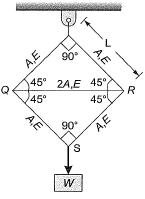Which one of the following sets gives the correct values of the force, stress and change in length of the horizontal member QR?    [2016 : 2 Marks, Set-II]
(a) Compressive force = 25 kN;
Stress = 250 kN/m2 ; Shortening = 0.0118 m
(b) Compressive force = 14.14 kN;
Stress = 141.4 kN/m2; Extension = 0.0118 m
(c) Compressive force = 100 kN;
Stress = 1000 kN/m2; Shortening = 0.0417 m
(d) Compressive force = 100 kN;
Stress = 1000 kN/m2; Extension = 0.0417 m
Ans.
(C)
Solution: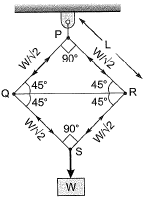Given data: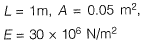Consider joint 'S’,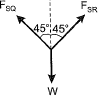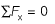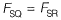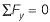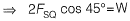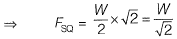As the truss is symmetrical,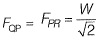(Tensile)
Now consider joint 'Q,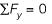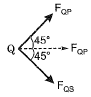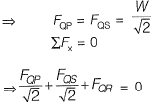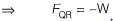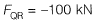(Compressive)
Stress in member OR,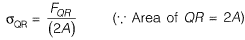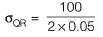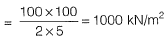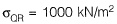As the member QR is subjecterd to compression, it will go under shortening.
∴ Shortening,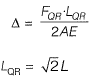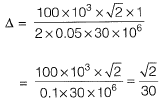∴ Δ = 0.0471 m

Q.5 A plane truss with applied loads is shown in the figure.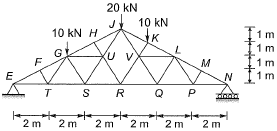The members which do not carry any force are    [2016 : 2 Marks, Set-I]
(a) FT, TG, HU, MP, PL
(b) ET, GS, UR, VR, QL
(c) FT, GS, HU, MP, QL
(d) MP, PL, HU, FT, UR
Ans.
(A)
Solution:
If there members meet at a joint and out of them are collinear, then non collinear member will carry zero force provided that there is no external load at the joint.
Use this statement to check the members with zero force.

Q.6 Consider the plane truss with load Pas shown in the figure. Let the horizontal and vertical reactions at the joint B be HB and VB, respectively and VC be vertical reaction at the joint C.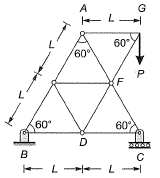Which one of the following sets gives the correct values of VB, Hand VC?   [2016 : 1 Mark, Set-I]
(a) VB = 0; HB = 0; VC = P
(b) VB = P/2; HB = 0; VC = P/2
(c) VB = P/2; HB = P (sin600); VC = P/2
(d) VB = P: HB = P (cos(600); VC = 0
Ans.
(A)
Solution: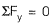⇒ VB + VC = P ...(i)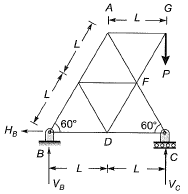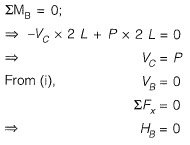Q.7 For the 2D truss with the applied loads shown below, the strain energy in the member XY is __________ kN-m. For member XY, assume AE = 30 kN, where A is cross-section area and E is the modulus of elasticity.    [2015 : 2 Marks, Set-I]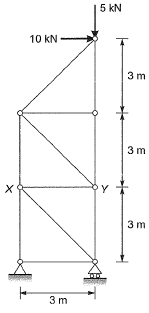Solution:

First calculating reactions,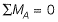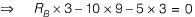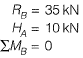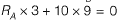RA = -30 kN
Let us cut a section 1-1 as shown in figure and consider the lower part.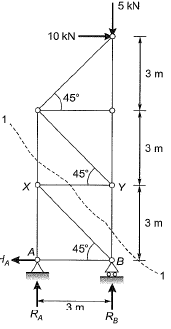Given AE = 30 kN and L = 3m,
Calculation for force Pin XY member.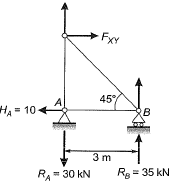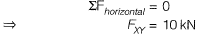Strain energy,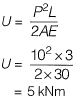Q.8 For the truss shown below, the member PQ is short by 3 mm. The magnitude of vertical displacement of joint R (in mm) is _______ .    [2014 : 2 Marks, Set-I]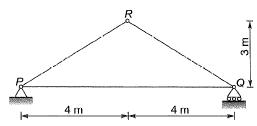Solution:
PQ is short by 3 mm
We have to find out vertical displacement of joint R in mm,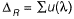Apply unit load at R as shown below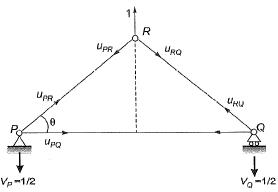Due to symmetric loading,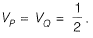Consider P:
∑V = 0,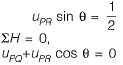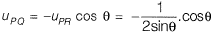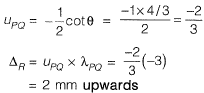Q.9 Mathematical idealization of a crane has three bars with their vertices arranged as shown in the figure with a load of 80 kN hanging vertically. The coordinates of the vertices are given in parentheses. The force in the member QR, FQR will be   [2014 : 2 Marks, Set-I]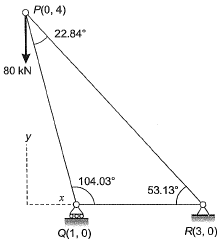(a) 30 kN Compressive
(b) 30 kN Tensile
(c) 50 kN Compressive
(d) 50 kN Tensile
Ans.
(A)
Solution: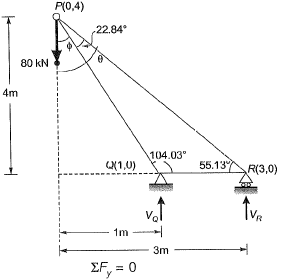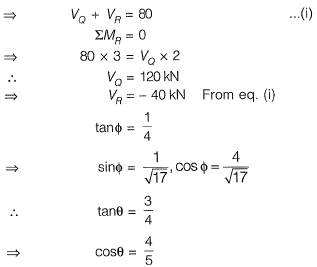Consider joint Q,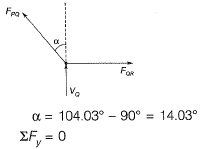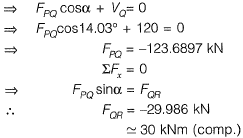Q.10 A uniform beam weighing 1800 N is supported at E & F by cable ABCD. Determine the tension force in segment AB at this cable (correct to 1 decimal place). Assume the cable ABCD, BE and CF are weightless    [2013 : 2 Marks]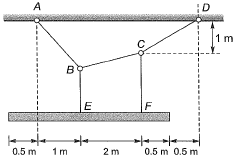Solution: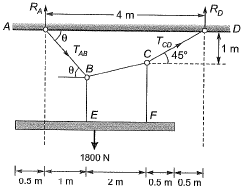RD x 4 = 1800 x 1.5
⇒ RD = 675 N
RA = 1800 - RD = 1125 N
At joint D,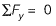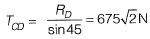Taking free body diagram as shown in figure,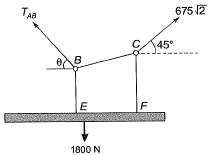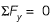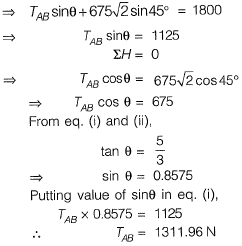Q.11 The pin-jointed 2-D truss is loaded with a horizontal force of 15 kN at joint S and another 15 kN vertical force at joint U as shown in figure. Find the force in member RS (in kN) and report your answer taking tension as +ve and compression as -ve.    [2013 : 1 Mark]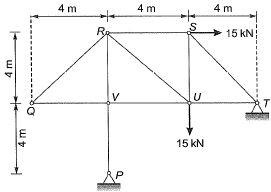Solution:

Take moment about V,Mv = 0,
⇒ RH, x 4 = 0
∴ RH = 0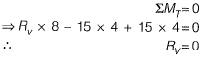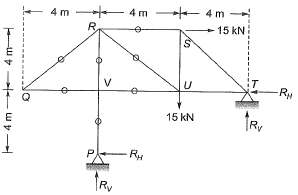We know that if two members meet at a. joint which are not collinear and also there is no external forces acting on that joint, then both members will carry zero forces.
∴ FQV - FQR = 0
Now, consider joint P,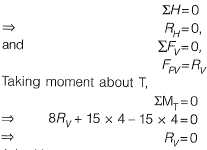Joint V,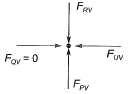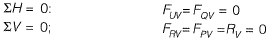Now, member RS and RU area non-collinear members meeting at a joint with no external force acting on the joint.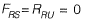Q.12 For the truss shown in the figure, the force in the member QR is    [2010 : 1 Mark]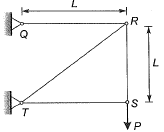(a) zero
(b) P/√2
(c) P
(d) √2 P
Ans.
(C)
Solution:
Using method of joints and considering joint S, we get,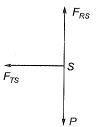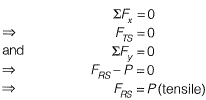Considering joint R, we get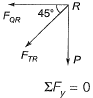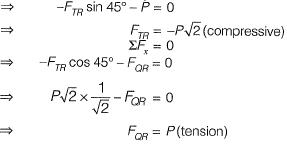Offer running on EduRev: Apply code STAYHOME200 to get INR 200 off on our premium plan EduRev Infinity!

69 docs

,

,

,

,

,

,

,

,

,

,

,

,

,

,

,

,

,

,

,

,

,

;